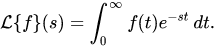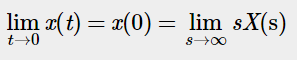### Laplace Transform

Laplace transform is an integral transform that converts a function in time domain into a function in frequency domain.
The real variable(t) is transformed into a complex variable(s) in the complex frequency domain i.e s-domain or s-plane.
Mathematical representation of laplace transform is given by:​​Initial Value Theorem
Initial value theorem in laplace transform allows us to examine the initial conditions of a system i.e initial values of the system parameters without the need to perform an inverse laplace transform.
Mathematical representation of Initial Value Theorem:Where, x(t) is the function in time domain, X(s) is the Laplace transform of the function and s is the complex variable in frequency domain.

Final Value Theorem
Final value theorem in laplace transform allows us to examine the final conditions of a system i.e final values of the system parameters without the need to perform an inverse laplace transform.
Mathematical representation of Final Value Theorem:Where, x(t) is the function in time domain, X(s) is the Laplace transform of the function and s is the complex variable in frequency domain.